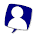Sunday, June 12, 2011

Significant Digits

By definition, significant digits are digits in a number that are meaningful. They are digits that contribute to the precision of a number. Consider these two measurements:
• 5 cm
• 5.000 cm
The two measurements may seem the same. However, the second one is much more precise because it has three more significant digits than the first one. For the first measurement, it can very well be 4.51 rounded up to 5, or it can be 5.49 rounded down to 5. On the other hand, the second measurement can only range from 4.995 to 5.005, and it has much more precision.

In the second measurement, the three 0s are significant because they contribute to the precision of the measurement.

So when is a digit insignificant? Well, the zeroes in this example are insignificant:
• 0.01 m
The zeroes in this number are insignificant because they are merely there to show that the number is less than one, and if you express this number is scientific notation, you'll get 1×10-2 metres: the zeroes will be gone!

Rules to determine whether a number is significant:

1. All non-zero digits (i.e.,1-9) are significant
2. All zeroes that are in between two non-zeroes digits are significant. For example, the zeroes in 5005 are significant
3. All leading zeroes are insignificant. For example, 0.0000000005 only have one significant digit (five)
4. If there is a decimal point in the number, the trailing zeroes are significant. For example, the zeroes in 5000.00 are all significant
5. If there is no decimal point in the number, the trailing zeroes may or may not be significant, and to be safe, we'll assume that they are insignificant. For example, the zeroes in the number 500 may or may not be significant, so we'll assume that they are not significant

Rounding:
Often in questions you have to be able to round your answer to a certain number of significant digits, and you basically round the numbers the way you usually do. For example, if you are rounding to two significant digits, 5.64 will be rounded to 5.6 and 7.77 will be rounded to 7.8.

There is one exception though. When there is only one digit that is to be omitted after rounding, and that digit happens to be a five, then you have to do one of the following:

• Round down if the last significant digit is an even number,
• Round up if the last significant digit is an even number

Okay, that is probably very hard to understand, so I will include an example. Say you have to round the numbers 8.65 and 7.75 to two significant digits. In both cases, only the 5s will have to be omitted after rounding. And to see whether to round up or not, you will look at the digits before the fives:

• 8.65
• 7.75

In the first case, the digit before the five is an even number (6), and therefore you need to round down to 8.6. On the other hand, the 7 in the second case is an odd number, and therefore you round up to 7.8.

Operations and Significant Digits
How do you know how many significant digits you need to round your answer to? Here are the rules
• With addition or subtraction, the result should have the same number of decimal places as the measurement that has the lowest number of decimal places. For example, if you are adding 16.7777 L to 222.1 L, the result should be rounded to one decimal place because 222.1 L only has one decimal place.

• With multiplication and division, the result should have the same number of significant digits as the measurement that has the lowest number of significant digits. For example, if you are multiplying 122 cm by 55 cm by 4455 cm, the result should have two significant digits because 55 only has two significant digits

1 comment:

1.Note: I know that significant digits are used in any type of calculations, not just calculations in chemistry. However, this is a topic that I have to review for my chemistry course...